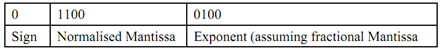## Explain about floating point representation, Computer Engineering

Assignment Help:

Q. Explain about Floating Point Representation?

Floating-point number representation includes two parts. The first part of number is a signed fixed-point number which is called mantissa and second part signifies decimal or binary point position and is called an Exponent. Mantissa can be an fraction or simply an integer. Please keep in mind that position of decimal or binary point is presumed and it's not a physical point thus wherever we are representing a point it's only assumed position.

A decimal + 12.34 in a distinctive floating point notation can be signified in any of subsequent two forms:

This number in any of above forms (if represented in BCD) needs 17 bits for mantissa (1 for sign and 4 every decimal digit as BCD) and 9 bits for exponent (1 for sign and 4 for every decimal digit as BCD). Please note the exponent denotes correct decimal location. In first case where exponent is +2 denotes that actual position of decimal point is two places to right of assumed position whereas exponent- 2 denotes that assumed position of point is two places in the direction of left of assumed position. The assumption of position of point is generally same in a computer resulting in a consistent computational environment.

Floating-point numbers are frequently represented in normalised forms. A floating point number whose mantissa doesn't comprise zero as most significant digit of number is considered to be in normalised form. For illustration a BCD mantissa + 370 that is 0 0011 0111 0000 is in normalised form since these leading zero's aren't part of a zero digit. On the other hand a binary number 0 01100 isn't in a normalised form. The normalised form of this number is:#### Describe the features of electronic banking, Question 1: The Banking Ac...

Question 1: The Banking Act provides for banking services through interactive electronic systems. (a) Describe the features of electronic banking. (b) Show the responsibi

#### Assembly directives and pseudo-ops, Assembly directives and pseudo-ops: ...

Assembly directives and pseudo-ops: Assembly directives are which instructions that executed by the assembler at assembly time, not by the CPU at run time. They can build the

#### Explain crossbar exchange with all call processing steps, Explain crossbar ...

Explain crossbar exchange, with all call processing steps and diagrams. The fundamental idea of crossbar switching is to give a matrix of n x m sets of contacts with only n + m

#### Instruction pipeline, example of branching take place in instructon pipelin...

example of branching take place in instructon pipeline

#### Objectives-interconnection network, Objectives After examining this uni...

Objectives After examining this unit, learner's will be able to understand discuss the importance and needs of interconnection network; explain the part of interconn

#### Define user space, Define user space? The system space is divided from ...

Define user space? The system space is divided from virtual address space in which the user application programs reside. The letter space is known as user space.

#### How online databases work, How Online Databases Work? An online or web-...

How Online Databases Work? An online or web-based database keeps data on a cloud of servers somewhere on the Internet, which is accessible by any authorized user with an Intern

#### What is software quality assuranc, Software QA includes the whole software ...

Software QA includes the whole software development PROCESS - improving and monitoring the process, making sure that any agreed-upon standards and processes are followed, and ensur

#### Example of processor arrangements, Q. Example of processor arrangements? ...

Q. Example of processor arrangements? !HPF\$ PROCESSORS P (10) This initiates a group of 10 abstract processors assigning them combined name P. !HPF\$ PROCESSORS Q (4, 4)

#### What are the digital certificates of hardware computations, What are the Di...

What are the Digital certificates of hardware computations Digital certificates are highly dependent on hardware computations, it is essential that mechanisms are evolved to i